# Height anomaly is the difference between the height of the surface of the common terrestrial ellipsoid, and the surface normal of the gravitational field of the Earth.

The curvature of the geoid can not be described mathematically. Therefore, the navigation problem solved on surfaces which have relatively simple mathematical description. These surfaces include spheroid and sphere.

The second approach to the physical surface of the Earth gives some approximation of an ellipsoid of revolution (spheroid). In solving navigation, geodetic and cartographic tasks often used approximation using an ellipsoid of revolution, which ignores the equatorial compression. This ellipsoid is characterized by:

• the location of its geometric center of the earth's center;

• orientation of the main axes;

• size large (equatorial) axis - a, small (polar) axis - b, polar compression - with, first eccentricity squared - e2 and the square of the second eccentricity - (еʹ)2.

Eccentricity is a value which characterizes the degree of elongation of the ellipse. The higher the semiaxes (a-b), the more the degree of elongation of the ellipse.

To calculate the flattening (c), the first and second squares eccentricities mathematical equations are used: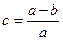;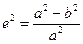;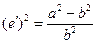Depending on the type of tasks surveying or navigation using such kinds of ellipsoids of rotation:

• common terrestrial ellipsoid;

• reference ellipsoid.

Common terrestrial ellipsoid – ellipsoid, the center and the equator coincide with the center of mass and the Earth's equator and is best approximates the geoid surface in planetary terms. An example of the MA can serve as a model of World Geodetic System WGS-84.

Q: What conditions should meet common terrestrial ellipsoid?

Center coincides with the center of the earth, b axis with an axis of rotation, the level is close to the geoid.

The deviation of the geoid from the ellipsoid (geoid undulations) is in the range of ≈-110m to ≈ + 80m. Now, based on satellite observations of the geopotential developed some models, for example, GRIM5 (Gravity Field Model), EGM96 (Earth Gravitational Model 1996). Geopotential model EGM96 recommended the International Earth Rotation Service for processing of geodetic and astrometric observations.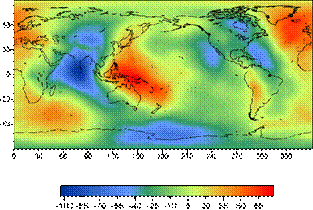The deviation of the geoid from the ellipsoid of rotation is much less than it would be if the continents, which have a lower density than the mantle, floating on an ellipsoidal Earth. Therefore, to explain the results of isostatic theory was proposed: the roots of continents, representing blocks of the Earth's crust, deep wedge into the denser mantle, and by the difference in densities of the crust and mantle is compensation for the gravity changes. Thus, changes geoid due to the state of the deep layers of the mantle, and is projected by the convective currents in the lower mantle. Therefore, the question of studying the form geoid, it changes over time paid much attention. Are currently being planned and a number of space projects, whose main task is to study the changes in the force of gravity (and hence the geoid) in space and time. The gravity anomalies will be identified with a relative error of ~ 10-8 and a spatial resolution of 100 km. Geoid height will be determined with an error of 1 cm.

- The need for WGS-84, causes.

- Where is use WGS-84?

- The main parameters of the WGS-84.

The use of global navigation systems require the creation of a global coordinate system. The main motivation to that served as the difference between the coordinates of the same point on the ground (for example, radio navigational point, the aerodrome reference point, etc.), in a variety of coordinate systems is 300-3000m. And this can be explained - in fact one and the same point on the Earth's surface is determined by the numerical values, the count of which was carried out by different starting time and with different orientation of the coordinate lines.

The use of accurate navigation coordinates with a variety of ways in aerial navigation will inevitably lead to a variety of aircraft flight paths, which can cause confusion in the locations and dominated by the lower level of safety, so there was a historical necessity in its creation and implementation. single global geodetic reference system.

Such a system is adopted to implement the ICAO in January 1998, World Geodetic System WGS-84 coordinates.

Implementation of this system involves the assignment of coordinates of the airport equipment and ways to navigate a route to a single system WGS-84.

WGS-84 is defined by a set of primary and secondary parameters.

The main parameters to describe the shape of the earth ellipsoid, its angular velocity and mass of the Earth, which is inherent in the common terrestrial ellipsoid.

Secondary parameters are defined full model of the gravitational field of the Earth. These parameters are used because the WGS-84 is used not only to determine the coordinates for geodesy and navigation, but the solution of problems of space systems, such as the organization and the satellite orbit correction system NAVSTAR (GPS).

The main parameters of the WGS-84:

• semi-major axis a = 6378137 m;

• semi-minor axis b = 6356752,314 m;

• compression ellipsoid = 3,35281066474 × 10-3;

However, from the point of view of common terrestrial Surveyors (middle earth) ellipsoid is not the best shape. It is a good approximation of the geoid, on average, in some parts of the surface of the ellipsoid of the geoid difference can be very large. Therefore, using geodetic techniques for different parts of the earth's surface were built local reference ellipsoid (in most developed countries before the start of the space age). As a rule, they are better approximate the geoid in some area than the common terrestrial ellipsoid, but the axis of the reference ellipsoid, in some cases, can be rotated about the axes of middle earth ellipsoid. In addition, the beginning of the axes may not coincide with the center of mass of the Earth.

The difference between the coordinate axes relative to the average of the measured or reference ellipsoid, be sure to take into account in science and in everyday life. This procedure is performed, for example, by landing aircraft, the coordinates of which are measured by GPS in the system WGS84, the airfield, the coordinates of which are defined relative to the axes of the local reference ellipsoid.

Reference ellipsoid (RE) - ellipsoid having the characteristics that best approximate the earth's surface in a given area.

QUESTION: What are the advantages of the reference ellipsoid of the common terrestrial ellipsoid, which characteristics of the reference ellipsoid does not coincide with the common terrestrial ellipsoid?

The best approximation of the earth's surface in the area, the centers RE and common terrestrial ellipsoid are not the same (and sometimes the direction of the semi-axes).

In order to achieve a minimum height squared deviations quasigeoid, databases modern electronic memory contain GPS-receivers are generally greater than 100 different Franz ellipsoids intended to achieve high-precision determinations on a particular site.

For example, recommended for use in the Commonwealth of Independent States Krasovskii reference ellipsoid provides accuracy determination of the height relative to the surface quasigeoid better than 40 m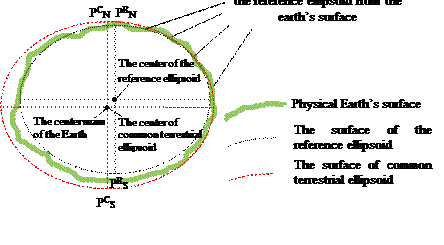The relative position of common terrestrial and the reference ellipsoid

on the physical surface of the Earth

 Physical Earth’s surface
 Oʹ
 A
 B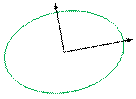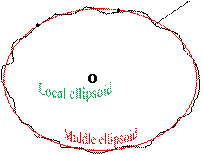The definition of common terrestrial ellipsoid and the reference ellipsoid for the area AB

For the territory of Ukraine and CIS countries used reference ellipsoid Krasousky.

a = 6378245 m b = 6356863 m c= 1/298, 3.

Sphere use for navigation. Since the compression is low, the shape of the Earth is not very different from the sphere. Therefore, when dealing with many navigation tasks that do not require high accuracy, the Earth is taken as the sphere. All basic calculations are made on the sphere.

Radius of the sphere equivalent in size Krasousky reference ellipsoid. It used to solve navigation problems.

Req = 6371 116 m

Дата добавления: 2015-06-10; просмотров: 1355; ЗАКАЗАТЬ НАПИСАНИЕ РАБОТЫ

Поиск по сайту:

При помощи поиска вы сможете найти нужную вам информацию.

Поделитесь с друзьями:

Если вам перенёс пользу информационный материал, или помог в учебе – поделитесь этим сайтом с друзьями и знакомыми.
helpiks.org - Хелпикс.Орг - 2014-2022 год. Материал сайта представляется для ознакомительного и учебного использования. | Поддержка
Генерация страницы за: 0.013 сек.